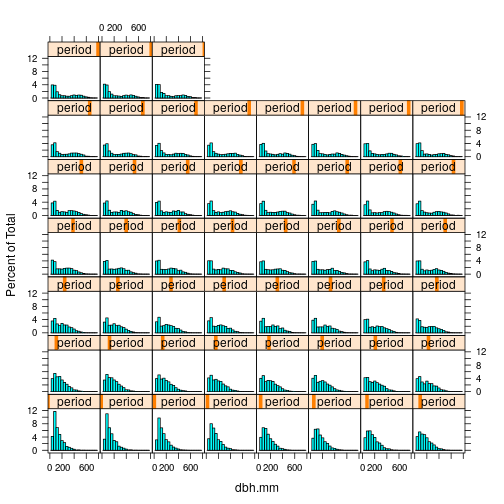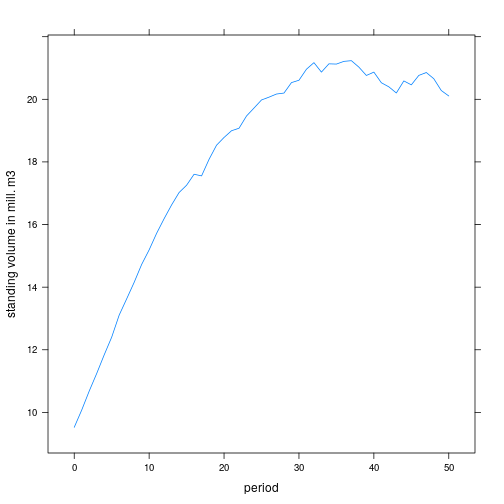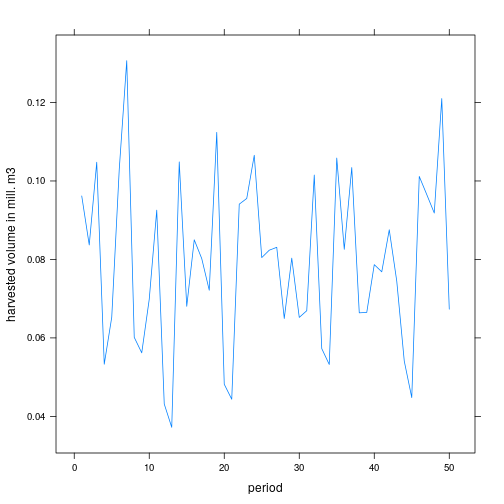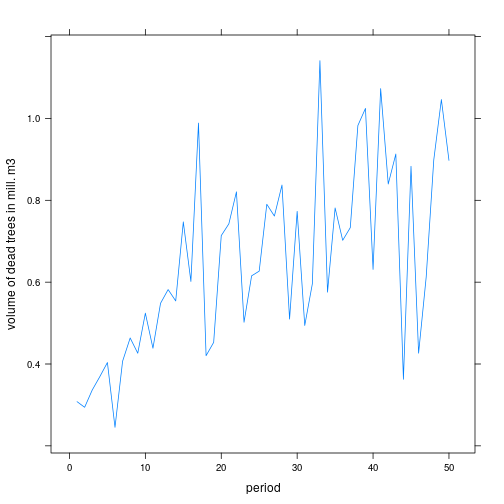title: “sitree: An Individual Tree Open Source Simulator” author: “Clara Antón Fernández” date: 31-07-2019 output: html_document: toc: true

# Introduction

The sitree package is designed to run single tree simulations where trees can be defined by diameter (or basal area), height, and tree species. sitree simulates birth, growth, and death of trees as well as management, if any. Functions can also be defined that affect characteristics of the stand (external modifiers), such as climate change, or fertilization. All variables that can be derived from the basic tree and stand characteristics (e.g. quadratic mean diameter, and tree volume) and that might be use for more than one function should be defined in a fn.prep.common.var, a function to prepare common variables. An example of a fn.prep.common.var is provided.

The easiest way to start with your own simulation is probably to modify the example functions provided (see the switching functions section of this vignette).

This tutorial gives examples on how you can use sitree to simulate individual tree growth, death, and harvest through time, and to compare the effects of changing functions (e.g. use a different mortality function) in the results of the simulation.

# Tree data classes and methods

There are two Reference Classes (RC) implemented in the sitree package, one for live trees (trList) and other for dead trees (trListDead).

• trList This class has two fields, data and nperiods. Under data basic information for each tree is stored (a unique stand ID plot.id, a unique tree ID treeid, and dbh and height for each period, dbh.mm, and height.dm). The nperiods field is an integer that stores the number of periods to be simulated.

• trListDead This class extends trList. DBH and heights measured while the tree was alive are stored under the data field. Also under this field information on how long the tree has been in the simulation can be found (yrs.sim). In this class the new field last.measurement stores the dimensions of the tree when it died or was removed. How these dimensions are calculated is defined on the dead.trees.growth function.

RC objects are mutable, they don't use R's usual copy-on-modify semantics, but are modified in place.

``````## Always bare in mind that RF trList and trListDead are modified in place
## Do not copy

Polygon <- setRefClass("Polygon", fields = c("sides"))
square <- Polygon\$new(sides = 4)

la <- function(a){
triangle <- square
triangle\$sides <- 3
return(a+2)
}

square\$sides
``````
``````##  4
``````
``````la(2)
``````
``````##  4
``````
``````square\$sides
``````
``````##  3
``````
``````## but if we do
square <- Polygon\$new(sides = 4)

la <- function(a){
triangle <- square\$copy()
triangle\$sides <- 3
return(a+2)
}

square\$sides
``````
``````##  4
``````
``````la(2)
``````
``````##  4
``````
``````## the object remains unchanged
square\$sides
``````
``````##  4
``````

Let\'s see now some examples of the methods implemented for these RF.

`````` require(sitree)
``````
``````## Loading required package: sitree
``````
`````` res <- sitree (tree.df   = tr,
stand.df  = fl,
functions = list(
fn.growth     = 'grow.dbhinc.hgtinc',
fn.mort       = 'mort.B2007',
fn.recr       = 'recr.BBG2008',
fn.management = 'management.prob',
fn.tree.removal = 'mng.tree.removal',
fn.modif      = NULL,
fn.prep.common.vars = 'prep.common.vars.fun'
),
n.periods = 5,
period.length = 5,
mng.options = NA,
fn.dbh.inc = "dbhi.BN2009",
fn.hgt.inc =  "height.korf",
species.spruce = c(1, 2, 3),
species.pine = c(10, 11, 20, 21, 29),
species.harw = c(30, 31),
fun.final.felling = "harv.prob",
fun.thinning      = "thin.prob",
'BN2009',
'BBG2008', 'SBA.m2.ha', 'spp','pr.spru.ba', 'QMD.cm',
per.vol.harv = 0.83
)

## getTrees(i, j)  -- obtains the information of the i trees, on the j periods,
## by default it selects all. It does not display it, it passes the value.
## It returns a list with elements plot.id, treeid, dbh.mm, height.dm, yrs.sim,
## tree.sp

get.some.trees <- res\$live\$getTrees(1:3, 2:5)

## extractTrees(i)  -- extracts the i trees, it removed the trees from the
## original object and it passes the information. It returns a list.

## addTrees(x) -- x should be a list

## last.time.alive. It checks when was the last DBH measured.
last.measurement = cbind(
, args = list(
growth = data.frame(dbh.inc.mm     = rep(3, 4),
hgt.inc.dm  = rep(8, 4)),
mort   = rep(TRUE, 4),
this.period = "t2")
),
),
nperiods = res\$live\$nperiods
)

## which in this case differs from the data stored under the last.measurement
## field because we have defined it artificially above as "t3"
lta
``````
``````##    4    5    6    7
## "t5" "t5" "t5" "t5"
``````
`````` new.dead.trees\$last.measurement\$found.dead
``````
``````##  t3 t3 t3 t3
## Levels: t3
``````
`````` ## But we can remove the data from the periods after it was found dead
## and now results do match
## last time it was alive was in "t2"
lta
``````
``````##    4    5    6    7
## "t2" "t2" "t2" "t2"
``````
`````` ## ant it was found dead in "t3"
``````
``````##  t3 t3 t3 t3
## Levels: t3
``````

# Taking a look at the results of the simulation

We first use the example functions available in the sitree package to run a 15 periods simulation.

``````set.seed(2017)
n.periods <- 50
res <- sitree (tree.df   = tr,
stand.df  = fl,
functions = list(
fn.growth     = 'grow.dbhinc.hgtinc',
fn.mort       = 'mort.B2007',
fn.recr       = 'recr.BBG2008',
fn.management = 'management.prob',
fn.tree.removal = 'mng.tree.removal',
fn.modif      = NULL,
fn.prep.common.vars = 'prep.common.vars.fun'
),
n.periods = n.periods,
period.length = 5,
mng.options = NA,
fn.dbh.inc = "dbhi.BN2009",
fn.hgt.inc =  "height.korf",
species.spruce = c(1, 2, 3),
species.pine = c(10, 11, 20, 21, 29),
species.harw = c(30, 31),
fun.final.felling = "harv.prob",
fun.thinning      = "thin.prob",
'BN2009',
'BBG2008', 'SBA.m2.ha', 'spp','pr.spru.ba', 'QMD.cm',
per.vol.harv = 0.83
)
``````

## dbh distribution through time

``````require(lattice)
``````
``````## Loading required package: lattice
``````
``````dbh.mm <- res\$live\$data\$dbh.mm
dbh.mm.short <- reshape(dbh.mm,
varying = paste0("t", 0:n.periods),
timevar = "period",
direction = "long", sep = "")
``````
``````##     period   t id
## 1.0      0 187  1
## 2.0      0 234  2
## 3.0      0 179  3
## 4.0      0  58  4
## 5.0      0 230  5
## 6.0      0 236  6
``````
``````dbh.mm.short\$t[dbh.mm.short\$t == 0] <- NA
histogram( ~ t | period, data = dbh.mm.short, plot.points = FALSE,
ref = TRUE, auto.key = list(space = "right"), xmin = 50,
xlab = "dbh.mm")
``````## Volume distribution through time

And since we already have the code to calculate volume we can easily plot the evolution of standing volume or harvested volume

``````vol <- data.frame(matrix(NA, ncol = n.periods+1, nrow = length(res\$plot.data\$plot.id)))
names(vol) <- paste0("t", 0:n.periods)
for (i.period in 0:n.periods){
sa <- prep.common.vars.fun (
tr = res\$live, fl= res\$plot.data,
i.period, this.period = paste0("t", i.period),
common.vars = NULL, vars.required = "vuprha.m3.ha",
period.length = 5 )

vol[, (i.period +1)] <- sa\$res\$vuprha.m3.ha
## This is volume per ha, if we prefer just m3 we multiply by ha2total
## ha2total is the number of ha represented by the plot
vol[, (i.period +1)] <- vol[, (i.period +1)] * sa\$fl\$ha2total
}
vol.m3.short <- reshape(vol,
varying = paste0("t", 0:n.periods),
timevar = "period",
direction = "long",
sep = "")
vol.m3.short\$t[vol.m3.short\$t == 0] <- NA
harv.total <- aggregate(t ~ period, data = vol.m3.short, FUN = sum)
xyplot( t/1e6 ~ period, data = harv.total, type = 'l',
ylab = "standing volume in mill. m3")
``````## Harvested volume

``````vol <- data.frame(matrix(NA, ncol = n.periods + 1,
nrow = length(res\$plot.data\$plot.id)))
## res\$removed\$data only contains the "history of the tree", but we need
## the dbh and height of the tree at harvest time
names(vol) <- paste0("t", 0:n.periods)
removed <- recover.last.measurement(res\$removed)

for (i.period in 0:n.periods){
sa <- prep.common.vars.fun (
tr = res\$removed,
fl = res\$plot.data,
i.period,
this.period = paste0("t", i.period),
common.vars = NULL,
vars.required = "vuprha.m3.ha",
period.length = 5 )

vol[, (i.period +1)] <- sa\$res\$vuprha.m3.ha
## This is volume per ha, if we prefer just m3.
vol[, (i.period +1)] <- vol[, (i.period +1)] * sa\$fl\$ha2total
}
names(vol) <- paste0(substr(names(vol), 1, 1), ".", substr(names(vol), 2, 3))

vol.m3.short <- reshape(vol,
varying = paste0("t.", 0:n.periods),
timevar = "period",
idvar = "id",
direction = "long"
)
vol.m3.short\$t[vol.m3.short\$t == 0] <- NA
harv.total <- aggregate(t ~ period, data = vol.m3.short, FUN = sum)
xyplot( t/1e6 ~ period, data = harv.total, type = 'l',
ylab = "harvested volume in mill. m3")
````````````vol <- data.frame(matrix(NA, ncol = n.periods + 1,
nrow = length(res\$plot.data\$plot.id)))
names(vol) <- paste0("t", 0:n.periods)

for (i.period in 0:n.periods){
sa <- prep.common.vars.fun (
i.period, this.period = paste0("t", i.period),
common.vars = NULL, vars.required = "vuprha.m3.ha",
period.length = 5 )

vol[, (i.period +1)] <- sa\$res\$vuprha.m3.ha
## This is volume per ha, if we prefer just m3.
vol[, (i.period +1)] <- vol[, (i.period +1)] * sa\$fl\$ha2total
}

names(vol) <- paste0(substr(names(vol), 1, 1), ".", substr(names(vol), 2, 3))
vol.m3.short <- reshape(vol,
varying = paste0("t.", 0:n.periods),
timevar = "period",
idvar = "id",
direction = "long"
)
``````
``````##     period t id
## 1.0      0 0  1
## 2.0      0 0  2
## 3.0      0 0  3
## 4.0      0 0  4
## 5.0      0 0  5
## 6.0      0 0  6
``````
``````vol.m3.short\$t[vol.m3.short\$t == 0] <- NA
harv.total <- aggregate(t ~ period, data = vol.m3.short, FUN = sum)
xyplot( t/1e6 ~ period, data = harv.total, type = 'l',
ylab = "volume of dead trees in mill. m3")
``````## Stand age

It is also interesting to look at the evolution of stand age

``````age <- res\$plot.data\$stand.age.years
age.short<- reshape(age,
varying = paste0("t", 0:(n.periods-1)),
timevar = "period",
idvar = "id",
direction = "long",
sep = ""
)
##histogram( ~ t | period, data = age.short, plot.points = FALSE,
##          ref = TRUE, auto.key = list(space = "right"), xmin = 50,
##          xlab = "stand age (in years)")
``````

# Switching functions

But the real power of sitree is how easily one can test new functions and its effects on important forestry variables. Let\'s see what happens when we switch mortality functions.

So far we have been using 'mort.B2007', Bollandås (2007) mortality equation. we will now try older Norwegian mortality functions. Those developed by Eid and Tuhus (2001).

``````ET2001 <- function (tr, fl, common.vars, this.period, ...)
{
if (!all(unique(common.vars\$spp) %in% c("spruce", "pine",
"birch", "other"))) {
message("spp should only contain values spruce, pine, birch, other")
}
dbh.mm <- tr\$data[["dbh.mm"]][, this.period]
p.functions <-
data.frame(a0 = c( 8.059,   8.4904, 4.892,  5.157),
b1 = c(-6.702, -14.266, -2.528, -7.3544),
b2 = c(-0.028,  -0.0462, 0,     -0.0199),
b3 = c(-0.0264, -0.0761, 0,     0 ),
b4 = c(-0.0132, -0.0761, 0,     0 )
)
rownames(p.functions) <- c("spruce", "pine", "birch", "other")

logit <- p.functions[common.vars\$spp, "a0"] +
p.functions[common.vars\$spp, "b1"] * (dbh.mm/10)^(-1) +
p.functions[common.vars\$spp, "b2"] * common.vars\$PBAL.m2.ha +
p.functions[common.vars\$spp, "b3"] * fl\$SI.m[common.vars\$i.stand] +
p.functions[common.vars\$spp, "b4"] * common.vars\$pr.spp.ba\$spru
mort.B <- 1- (1/(1 + exp(-logit)))
mort <- ifelse(mort.B >= runif(length(tr\$data[["plot.id"]]),
0, 1), TRUE, FALSE)
return(mort)
}
``````

Let\'s take a look at standing volume, but first, since there are several stochastic algorithms in the functions selected to run the simulation, we will calculate averages for several runs. The code below can be easily run in parallel but we will keep it in a simmple loop for the shake of clarity.

First, let\'s run it with mort.B2007

``````set.seed(2017)
n.periods <- 10
i.max <- 50
vol.1 <- data.frame(matrix(NA, ncol = n.periods, nrow = i.max))
names(vol.1) <- paste0("t", 0:n.periods)

for (i in (1:i.max)){
print(i)
res1 <- sitree (tree.df   = tr,
stand.df  = fl,
functions = list(
fn.growth     = 'grow.dbhinc.hgtinc',
fn.mort       = 'mort.B2007',
fn.recr       = 'recr.BBG2008',
fn.management = 'management.prob',
fn.tree.removal = 'mng.tree.removal',
fn.modif      = NULL,
fn.prep.common.vars = 'prep.common.vars.fun'
),
n.periods = n.periods,
period.length = 5,
mng.options = NA,
fn.dbh.inc = "dbhi.BN2009",
fn.hgt.inc =  "height.korf",
species.spruce = c(1, 2, 3),
species.pine = c(10, 11, 20, 21, 29),
species.harw = c(30, 31),
fun.final.felling = "harv.prob",
fun.thinning      = "thin.prob",
'BN2009',
'BBG2008', 'SBA.m2.ha', 'spp','pr.spru.ba', 'QMD.cm',
per.vol.harv = 0.83
)

for (i.period in 0:n.periods){
sa <- prep.common.vars.fun (
tr = res1\$live, fl= res1\$plot.data,
i.period, this.period = paste0("t", i.period),
common.vars = NULL, vars.required = "vuprha.m3.ha",
period.length = 5 )

vol.1[i, (i.period +1)] <- sum(sa\$res\$vuprha.m3.ha * sa\$fl\$ha2total )

}
}
``````

And now with ET2001.

``````vol.2 <- data.frame(matrix(NA, ncol = n.periods, nrow = i.max))
names(vol.2) <- paste0("t", 0:n.periods)

for (i in (1:i.max)){
print(i)

res2 <- sitree (tree.df   = tr,
stand.df  = fl,
functions = list(
fn.growth     = 'grow.dbhinc.hgtinc',
fn.mort       = 'ET2001',
fn.recr       = 'recr.BBG2008',
fn.management = 'management.prob',
fn.tree.removal = 'mng.tree.removal',
fn.modif      = NULL,
fn.prep.common.vars = 'prep.common.vars.fun'
),
n.periods = n.periods,
period.length = 5,
mng.options = NA,
fn.dbh.inc = "dbhi.BN2009",
fn.hgt.inc =  "height.korf",
species.spruce = c(1, 2, 3),
species.pine = c(10, 11, 20, 21, 29),
species.harw = c(30, 31),
fun.final.felling = "harv.prob",
fun.thinning      = "thin.prob",
'BN2009','PBAL.m2.ha',
'BBG2008', 'SBA.m2.ha', 'spp','pr.spru.ba', 'QMD.cm',
per.vol.harv = 0.83
)

for (i.period in 0:n.periods){
sa <- prep.common.vars.fun (
tr = res1\$live, fl= res1\$plot.data,
i.period, this.period = paste0("t", i.period),
common.vars = NULL, vars.required = "vuprha.m3.ha",
period.length = 5 )

vol.2[i, (i.period +1)] <- sum(sa\$res\$vuprha.m3.ha * sa\$fl\$ha2total )

}
}
## During the five periods, there is actually not that much of a difference
colMeans(vol.1)/1e6
colMeans(vol.2)/1e6
``````

# References

Bollandsås, O. 2007 Uneven-aged Forestry in Norway: Inventory and Management Models. [Ås, Norway]: Norwegian University of Life Sciences, Department of Ecology and Natural Resource Management.

Eid, T., and Tuhus E. 2001 Models for Individual Tree Mortality in Norway. Forest Ecology and Management 154 (½): 69–84.